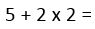# 11-Plus Exam Illustrations - Maths Quiz - Order in Sums - BIDMAS (Questions)

To get to grips with BIDMAS (also known as BODMAS), have a read of our in-depth article on BODMAS/BIDMAS.

The simple sum gets a lot harder when you have multiple parts to it. Look at this example:The answer, depending on how you do it, is 14 (5+2 is 7, x2 is 14) or 9 (2x2 is 4, and then add that to 5).

Children get taught BIDMAS or BODMAS as a way to work out which part of a sum to do first. The acronyms mean:

 B brackets I / O index / order D divide M multiply A add S subtract

If you follow the rule, you should carry out a sum in brackets before anything else. Then look at index / order (that means numbers 'to the power...'; think of three squared (32) as three to the power two). Next you would do the division, then multiplication, addition and finally subtraction.

However, in a test this may not be expected - it can be really tricky to know. I have seen 11+ papers which simply expect a child to run through a list of operations in order, regardless of BIDMAS. Bizarrely, these can appear on verbal reasoning papers too.

If you see '3 + 4 x 5 =' you may expect the answer to be 35 as it is 3+4 multiplied by 5. Technically, a mathematician would say it's wrong - you need to do the multiplication first and therefore the answer is 23. Very confusing - if in doubt, look to see whether there are any clues. Is there a sample sum done following BIDMAS or not? Does it mention 'be careful to carry out operations in the correct order'? Are there multiple choice options and only one of the 'correct' answers is on there? If so, apply the same reasoning to all similar questions.

Generally I tell a child to ignore BIDMAS (at least, the ...DMAS part) unless there are clues suggesting you should use it. However, at secondary school it will be taught from the outset and you will be expected to use it accordingly. Brackets are ALWAYS to be carried out before anything else though.